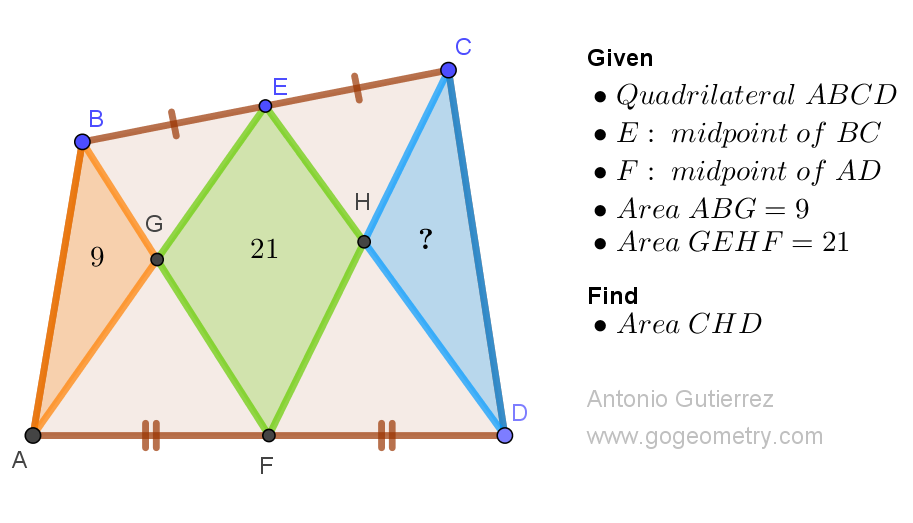# Geometry Problem 1536: Discover the Power of Midpoints: Solving for Missing Areas in Quadrilaterals

Given a quadrilateral ABCD with midpoints E and F on sides BC and AD, respectively, AE intersects BF at G, and DE intersects CF at H. If the area of triangle ABG is 9 and the area of the quadrilateral GEHF is 21, find the area of triangle CHD.Problem 1536
Midpoints, lines meet,